﻿ Kakuro решение
 ВходРегистрация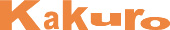# Kakuro решение

The task in Kakuro puzzles is to fill all empty squares using numbers 1 to 9 so the sum of each horizontal block equals the clue on its left, and the sum of each vertical block equals the clue on its top. In addition, no number may be used in the same block more than once. The best way to learn how to solve Kakuro puzzles is to see how a puzzle is solved from beginning to end.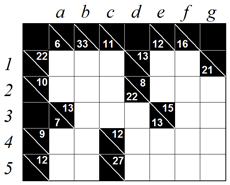## Step 1

Kakuro puzzles are all about special number combinations. Let’s examine the 22-in-three block in row 1. The only possible combinations are 5+8+9 and 6+7+9. However, square a1 must be smaller than 6 because of the 6-in-two block in column a. Therefore the only number possible in square a1 is 5. Completing the vertical 6-in-two block in column a is now straightforward, since only 1 can be placed in square a2.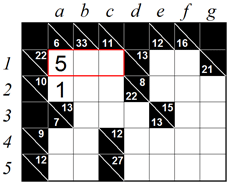## Step 2

From step 1 we know squares b1 and c1 must contain 8 and 9, though we don’t yet know in which order. Let’s look at the 11-in-three block in column c. If the 9 is in square c1 then squares c2 and c3 must both contain 1, which is not allowed. This means square c1 must be 8 and square b1 must be 9.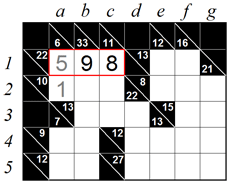## Step 3

We are now left with two empty squares in column c which must sum up to 3. The only combination is 1+2, but again we don’t know in which order. However, square a2 already contains 1 so the number in square c2 must be 2. Completing column c and row 2 is now straightforward.## Step 4

Let’s look at the vertical 16-in-five block in column f. This is a magic block because the only five-number combination that sums up to 16 is 1+2+3+4+6. So we know all numbers in this block but we don’t know in which order they appear. Now let’s examine the horizontal 15-in-two block in row 3. There are only two combinations possible: 6+9 and 7+8. Since square f3 is the crossing point, it must contain 6 which is the only common number for both blocks. This leads to placing a 9 in square g3.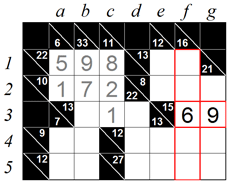## Step 5

Looking at the 13-in-two block in row 1 we see the only allowed combinations are 4+9, 5+8 and 6+7. However, this block crosses the 16-in-five block in column f, which is still missing 1, 2, 3 and 4. Therefore the only common number for square f1 is 4. We can now complete the 13-in-two block with 9 in square e1, and the 12-in-two block with 3 in square e2.## Step 6

The 8-in-three block in row 2 still has two empty squares which sum up to 5. There are two possible combinations: 1+4 and 2+3, but the 2+3 is not allowed because this block already contains 3. In addition, 4 can’t be in square f2 because the 16-in-five block in column f already contains 4.

This means that Square f2 must be 1, and Square g2 must be 4.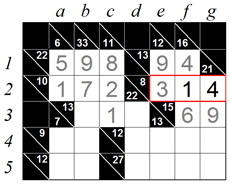## Step 7

The 16-in-five block in column f now misses only 2 and 3. Let’s take a close look at the 27-in-four block in row 5. If square f5 contained 2, the remaining three squares must add up to 25. But this is not possible because the largest sum of three squares is 7+8+9=24. Therefore square f5 is 3 and square f4 is 2.## Step 8

We now come across a special situation which occurs in the right hand side of the puzzle. If we make a vertical sum of columns d, e, f and g we get 22+12+13+16+21=84. If we now make a horizontal sum of the same area, excluding square d3, we get 13+8+15+12+27=75. This means square d3 is responsible for the difference and therefore must be 84-75=9. To complete this block, a 3 is placed in square b3.## Step 9

Let’s go back to the 27-in-four block in row 5 which has three empty squares that sum up to 24. These three empty squares now form their own magic block because the only possible combination is 7+8+9 in some order. However, the 9 can’t be in square d5 or g5 because each of those vertical blocks already has 9. So the only place left for 9 is square e5. We can now also place 4 in square e4 by simple calculation.## Step 10

The 12-in-four block in row 4 has two empty squares that sum up to 6. The possible combinations are 2+4 and 1+5 but obviously only 1+5 is allowed. We now need to determine which square is 1 and which is 5. If we try to place 1 in square d4 we immediately see square d5 will be larger than 9. Therefore square d4 must be 5 and square g4 must be 1. We can now complete columns d and g with 8 in square d5 and 7 in square g5.## Step 11

Finally let’s examine the 33-in-five block in column b. There are two empty squares that sum up to 14 so the only possible combinations are 5+9 and 6+8. However, 9 is already used in column b so we’re left with 6+8. If we choose 6 for square b5 then square a5 would also have to be 6 leaving 8 as the only possible candidate for square b5. Completing the remaining squares b4, a4 and a5 is now straightforward.